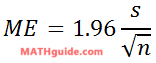Mean, Standard Deviation, and Margin of Error
View the Lesson | MATHguide homepage Updated June 22nd, 2023

Given: Sentences were chosen at random from Anne H. Ehrlich and Paul R. Ehrlich's book The Population Explosion. The number of words in the sentences were counted. This is the result.

 13 11 3 17 8 11 19 6 11 15 20 10 23 9 23 15 3 7 11 6 15 12 14 19
Problem:Use the data above to: a) determine the mean and sample standard deviation, b) calculate a 95% margin of error, c) determine the respective confidence interval.Mean (x̄) =

Sample Standard Deviation (s) =

Margin of Error =

The confidence interval is [, ]